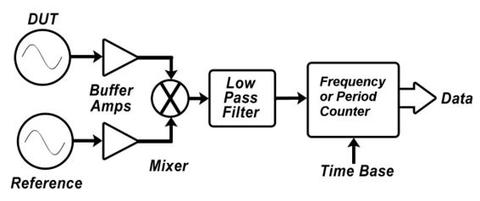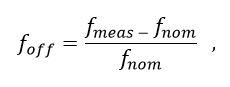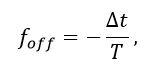An official website of the United States governmentOfficial websites use .gov
A .gov website belongs to an official government organization in the United States.Secure .gov websites use HTTPS
A lock ( ) or https:// means you've safely connected to the .gov website. Share sensitive information only on official, secure websites.

# Time and Frequency from A to Z, F

## Share

Femtosecond (fs)

A unit of time that represents one quadrillionth of a second (10-15 s).

Flicker Noise

A type of low frequency noise where the power spectral density is inversely proportional to the frequency. For this reason, it is sometimes referred to as 1/f noise.

Frequency

The rate of a repetitive event. If T is the period of a repetitive event, then the frequency f is its reciprocal, 1/T. Conversely, the period is the reciprocal of the frequency, T = 1 / f. Because the period is a time interval expressed in seconds (s), it is easy to see the close relationship between time interval and frequency. The standard unit for frequency is the hertz (Hz), defined as the number of events or cycles per second. The frequency of electrical signals is often measured in multiples of hertz, including kilohertz (kHz), megahertz (MHz), or gigahertz (GHz).

Frequency Accuracy

The degree of conformity of a measured or calculated frequency to its definition. Because  accuracy is related to the offset from an ideal value, frequency accuracy is usually stated in terms of the frequency offset.

Frequency Comb

The accurate measurement of optical frequencies had historically been difficult, involving large and complex chains of frequency-doubled and frequency-mixed lasers. This changed in the early part of the twenty-first century, with the invention of the self-referenced femtosecond laser frequency comb. These devices, which are now sold commercially, have made the linkage of optical to microwave frequencies, or optical to optical frequencies, relatively simple.

A self-referenced femtosecond laser frequency comb generates a series of discrete, equally frequency-spaced modes. The mode spacing, frep, is given by the repetition rate of a mode-locked laser, which is typically around 1 GHz. The frequency of an individual mode can be expressed as f(m) = fo + m × frep, where m is an integer. The frequency offset fo is measured by a method called self-referencing. An optical standard can be compared to a conventional frequency standard by referencing the comb to a cesium standard or a GPSDO and then measuring the heterodyne beat frequency fbeat between the optical standard and the nearest tooth of the comb. This will lead to determination of the frequency of the optical standard in terms of fo, frep, and fbeat, if the integer m is known. Frequency combs also allow the ratio of two optical frequencies to be compared without being limited by the accuracy of conventional microwave standards. This has already resulted in frequency comb comparisons of optical standards with relative frequency differences of parts in 1019.

Frequency Counter

An electronic instrument or circuit that measures and displays the frequency of an incoming signal. Frequency counters are the most common instruments used to measure frequency.  The measurements are referenced to the counter’s time base oscillator, which is usually a quartz oscillator of unknown accuracy (typically no better than 1 × 10-8).  For this reason, the best available oscillator should be connected to the counter’s external time base input. The smallest frequency offset that a frequency counter can detect with a single reading will be limited by the number of digits on the counter’s display. For example, a 10-digit counter cannot detect a frequency change smaller than 1 × 10-9 without averaging when measuring a 10 MHz signal, but a 12-digit counter can reduce this value by two orders of magnitude to 1 × 10-11

Frequency Divider

An electronic instrument or circuit that converts an incoming signal to a lower frequency by removing a fixed number of cycles or pulses from the signal. For example, a circuit that divides by 1000 can accept a 1 MHz signal as an input, and produce a 1 kHz signal as an output. Dividers are commonly used to convert a standard frequency standard such as 5 MHz or 10 MHz to a 1 Hz signal that can be synchronized to UTC for timing applications.

Frequency Domain

The measurement domain where voltage and power are measured as functions of frequency.  A spectrum analyzer can analyze signals in the frequency domain by separating signals into their frequency components and displaying the power level at each frequency. An ideal sine wave (perfect frequency) appears as a spectral line of zero bandwidth in the frequency domain. Real sine wave outputs are always noisy, so the spectral lines have a finite bandwidth, as shown in the graphic. Noise is usually present over a wide band of frequencies.Frequency Drift

An undesired progressive change in frequency with time.  Frequency drift can be caused by instability in the oscillator and environmental changes, although it often hard to distinguish between drift and oscillator aging. Frequency drift may be in either direction (resulting in a higher or lower frequency) and is not necessarily linear.

Frequency Mixer

An electronic instrument or circuit that accepts two input signals at two different frequencies, and produces an output frequency (called the beat frequency or difference frequency), that is equal to the difference of the two inputs. Frequency mixers are commonly employed in frequency measurement systems to convert a high frequency to a low frequency, and to obtain more measurement resolution. For example, a 5 MHz signal might be mixed with a 5,000,010 Hz signal. Measuring the 10 Hz beat frequency with a frequency counter (as opposed to the 5 MHz) allows the detection of smaller frequency changes, as shown in the graphic.Frequency Multiplier

An electronic instrument or circuit that converts an incoming signal to a higher frequency by adding a fixed multiple of cycles or pulses to the signal. For example, a circuit that multiplies by 10 could accept a 1 MHz signal as an input, and produce a 10 MHz signal as an output.

Frequency Offset

The difference between a measured frequency and an ideal frequency with zero uncertainty. This ideal frequency is called the nominal frequency.

Frequency offset can be measured in either the frequency domain or the time domain. A simple frequency domain measurement involves directly counting and displaying the output frequency of the device under test with a frequency counter. The frequency offset is calculated as:where fmeas is the reading from the frequency counter, and fnom is the specified output frequency of the device under test.

Frequency offset measurements in the time domain involve measuring the time difference between the device under test and the reference. The time interval measurements can be made with an oscilloscope or a time interval counter. If at least two time interval measurements are made, we can estimate frequency offset aswhere ∆t is the difference between time interval measurements (phase difference), and T is the measurement period.

Frequency offset is usually expressed as a dimensionless number such as 1 × 10-10, since the quantities being measured are typically very small. Using dimensionless values does not require knowledge of the nominal frequency. However, they can be converted to units of frequency (Hz) if the nominal frequency is known. To illustrate this, consider a device with a nominal frequency of 5 MHz and a frequency offset of +1.16 × 10-11. To find the frequency offset in hertz, multiply the nominal frequency by the offset:

(5 x 106) (+1.16 x 10-11) = 5.80 x 10-5 = +0.0000580 Hz

Then, add the frequency offset to the nominal frequency to get the actual frequency:

5,000,000 Hz + 0.0000580 Hz = 5,000,000.0000580 Hz

Frequency Shift

A sudden change in the frequency of a signal.

Frequency Stability

The degree to which an oscillating signal produces the same frequency for a specified interval of time. It is important to note the time interval; some devices have good short-term stability, others have good long-term stability. Stability doesn't tell us whether the frequency of a signal is right or wrong, it only indicates whether that frequency stays the same. The Allan deviation is the most common metric used to estimate frequency stability, but a number of similar statistics are also used.

Frequency Standard

An oscillator (usually an atomic oscillator) that is used as a reference source for frequency measurements. The frequency standard in a calibration laboratory is generally a cesium oscillator, a rubidium oscillator, or a GPS disciplined oscillator.  The current frequency standard for the United States is a cesium fountain oscillator named NIST-F2.

Frequency Synthesizer

An electronic device or circuit that can produce a wide range of user selectable output frequencies. In order to produce a wide range of frequencies, frequency synthesizers typically contain several components, including frequency dividers, frequency multipliers, and phase locked loops.

Created May 12, 2010, Updated September 26, 2016